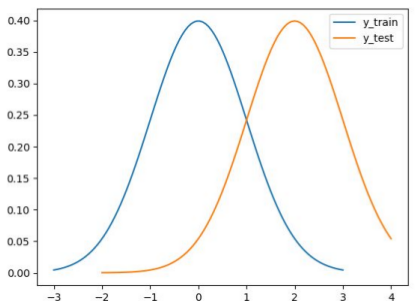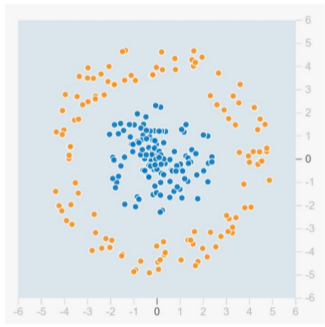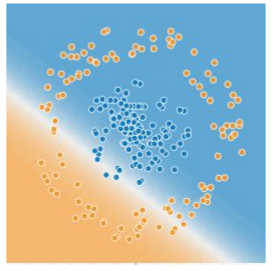Phone

+919997782184

Email

support@roboticswithpython.com

Geeks of Coding

Home Forums Assignment NPTEL_ Practical Machine learning with tensor flow Practical Machine Learning with Tensorflow – Assignment 2

• Author
Posts
• #384

i) Mini batch gradient descent is implemented by dividing the dataset into small batches and processing all the batches parallely (vectorization).
ii) Training one epoch of mini batch gradient descent is faster than training one epoch of batch gradient descent.
Answer- Both (i) and (ii) are true.

2)- We want to perform regression over training data (x_train, y_train) and test the model on (x_test, y_test). Suppose y_train and y_test
come from the following distributions:After training the model, when we evaluate it on the test data, which of the following situation is more likely to occur?
Answer- `Low error when y_test=2 and high error when y_test=-2`

3)- Suppose we want to predict the amount of rainfall R (which has factors like seasons, trends, time of day, temperature etc.) given input data X = {x1 , x2 , x3 , x4 }. We have 1000 training data points. We train the data using the following models: (i) R = a ​ sin(​ bX​ ) + ​ c sin(​ dX​ ) + f(​ X) where f() is a polynomial function, (ii) NN(x) where NN() is a neural network with 10 hidden layers​ ​ with 4 units each (where a, b, c, … are the parameters of the model) and (iii) R = aX^3 + bX + c. Select the option that correctly identifies the model that under its, just fits, and overfits respectively:

4)- Given the following confusion matrix for a classification task with three classes (A, B and C), which of the following is correct:
Ground Truth/Predict—————A————–B————C
A———————————0————–0————-2

B———————————1————–3————-1

C———————————0————–0————-3

Answer- `\Precision(B) = 1.0, Precision(C) = 0.5`

5)- From the confusion matrix in Q4, select the correct value of f1_score(B)

Answer-`0.75`

6)- What is the range of average f1-score (previous question)?

Hint: Use the total number of ground-truth class of each class to compute avg f1-score.

Answer- `0.4 to 0.59`

7)- Which metric is foolproof while evaluating the classification task mentioned in the previous question?

Answer- `F1 Score`

8)-If you are given the following data for classification (use tensorflow playground):Select the set of inputs that would be enough for a single layer (Input -> Output) neural network for best performance. (Assume tanh activation)
Answer- `X1^2, X2^2`

9)- Suppose we provide x1, x2 as inputs for the above case, and add one hidden layer, what is the minimum number of neurons required to get circular (or at least polyhedral) decision boundary?
Answer-`3`

10)- Suppose we provide x1, x2 as inputs in Q9, add one hidden layer and train the model to learn the optimal decision boundary, what can the outputs of the neurons in the hidden layer look like?11)- Suppose we provide x1, x2 as inputs in Q9, add one hidden layer with 4 neurons and train the model, will the model be able to learn the optimal decision boundary with all four activation functions (ReLU, Sigmoid, Tanh, Linear)?</div>
Answer- `No`

12)- Let f(x, y) = g(w1 x + w2 y + b), where x and y are binary inputs i.e., x, y ∊ {0, 1}; w1 , w2 and b are the parameters and let g(z) be Relu activation. This function can fit on:
Answer- `NOT(x), AND(x, y), OR(x, y)`